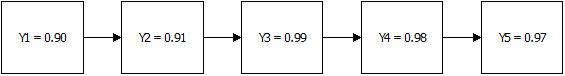# Six Sigma Metrics

## Six Sigma Metrics

Six Sigma practitioners use many Six Sigma metrics and/or measures of performance. In addition to the ones we will cover here, several others (Sigma level, Cp, Cpk, Pp, Ppk, takt time, cycle time, utilization, etc.) will be covered in other articles. The Six Sigma metrics of interest in the Define phase are:

• Defects per Unit
• Defects per Million Opportunities
• Yield
• Rolled Throughput Yield
##### Defect per Unit (DPU)

DPU or Defects per Unit, is the basis for calculating DPMO and RTY, which we will cover in the next couple sections. DPU is found by dividing total defects by total units.

• DPU = D/U

For example, if a process step produces 65 defects for every 600 units, then:

• DPU = 65/600 = 0.1083 or 10.9%.
##### Defect per Million Opportunities (DPMO)

DPMO, or Defects per Million Opportunities, is one of the few important Six Sigma metrics you should get comfortable with if you are associated with Six Sigma. Remember that Six Sigma represents 3.4 defects per million opportunities?

To understand DPMO, it is best if you first understand both the
nomenclature and the nuances, such as the difference between defect and defective.

Nomenclature:

• Defects = D
• Unit = U
• Opportunity to have a defect = O

To properly discuss DPMO, we must first explore the differences between “defects” and “defective.”

Defective

Defective suggests that the value or function of the entire unit or product has been compromised. Defective items will always have at least one defect. Typically, however, it takes multiple defects and/or critical defects to cause an item to be defective.

Defect

A defect is an error, mistake, flaw, fault, or imperfection that reduces a product's or unit's value. A single defect may or may not render the product or unit “defective,” depending on the customer's specifications.

To summarize, a defect means that part of a unit is bad. Defective means that the whole unit is bad.

Now let us define “opportunities” to understand Defects per Million Opportunities (DPMO) fully.

Opportunities

Opportunities are the total number of possible defects. Therefore, if a unit has six possible defects, each unit produced equals six defect opportunities. If we produce 100 units, there are 600 defect opportunities (100 units × 6 opportunities/unit).

• DPMO = [D / (U x O)] x 1,000,000

For example, let us assume there are six defect opportunities per unit and there is an average
of 4 defects every 100 units.

• Opportunities = 6 × 100 = 600
• Defect rate = 4/600
• DPMO = 4/600 × 1,000,000 = 6,667

What is the reason or significance of 1,000,000? Converting defect rates to a “per million” value becomes necessary when your process performance approaches Six Sigma. When this happens, the number of defects shrinks to virtually nothing. If you recall from the "What is Six Sigma” section, Six Sigma is equivalent to 3.4 defects per million opportunities. Using 1,000,000 opportunities as the barometer, we have the resolution in our measurement to count defects up to Six Sigma.

##### Rolled Throughput Yield (RTY)

Rolled Throughput Yield (RTY) is a process performance measure that provides insight into the cumulative effects of an entire process. RTY measures the yield for each of several process steps and provides the probability that a unit will come through that process defect-free.

RTY allows us to expose the “hidden factory” by providing visibility into the yield of each process step. This helps us identify the poorest performing process steps and gives us clues into where to look to find the most impactful process improvement opportunities.

Calculating RTY

RTY is found by multiplying the yields of each process step. Let us take the five-step process
below and calculate the RTY (the yield or percent of units that get through without a defect)
using the multiplication method mentioned above.The RTY calculation for the above figure is: RTY = 0.90 × 0.91 × 0.99 × 0.98 × 0.97 = 0.77

Therefore, RTY = 77%. In this example, 77% of units get through all five process steps without a defect. You may have noticed that to calculate RTY we must determine the yield for each process step. Before we get into calculating yield, there are a few abbreviations that need to be
declared.

• Defects = D
• Unit = U
• Defects per Unit = DPU
• Yield = Y
• e = 2.71828 (mathematical constant)

Calculating Yield

The yield of a process step is the success rate or the probability that the process step produces no defects. To calculate yield, we need to know DPU, and then we can apply it to the yield equation below.

Y = e-DPU

For example, let's assume a process step has a DPU of 0.109 (65/598).

Y = 2.718−0.109 = 0.8967
Rounded, Y = 90%.

In this example, DPU (defects per unit) is 0.109 (65 defects/598 units). Plugging in the DPU and using the mathematical constant for e (2.718), the result is 0.8967, or 90%, when rounded.

Estimating Yield

Instead of using the equation provided earlier, there is a simpler way to calculate yield using yield estimation: It is possible to “estimate” yield by taking the inverse of DPU or simply subtracting DPU from 1.

Yield Estimation = 1 – DPU

Estimating Yield in this way assumes max DPU is 1.